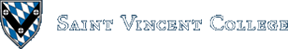Computing & Information Systems    DepartmentSearch CIS Site      Tutorials

## Software Design Using C++

### Array Basics

An array is used to hold a sequence of data items, all of the same type. The data items are indexed, starting with index 0. In C++, all array indexing begins at 0. An array of 5 characters, for example, could be set up as follows:

 `````` char CharArray; ``````

This array would be indexed 0, 1, 2, 3, 4. We could put the character 'R', for example, into the array location at index 0 by using the following:

 `````` CharArray = 'R'; ``````

We could put data at the other array locations in a similar manner. In fact, we could input data into `CharArray`, print out `CharArray`, etc. The array can be pictured as shown below (with additional characters filled in at the other array slots):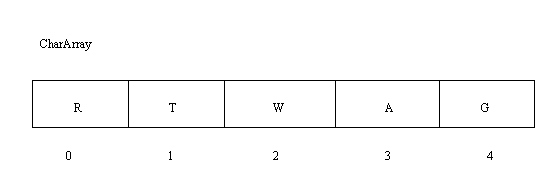### Using Loops with Arrays

When placing data into an array, printing data from an array, or processing array data in some manner, one typically needs a loop to repeat the same operation on each data item. The arrays.cpp example shows the use of a `for` loop to enter data into the array and another `for` loop to print the array's contents.

Let's look at some of the details of this program. Note that it sets up a constant called `ArrayMax` for the maximum number of items that can be placed in the array, as well as a type name, `ArrayType`, for the array. Here the typedef is used to define `ArrayType` to be a type name for any array of `ArrayMax` integers.

In the `main` function you see that `MyArray` is declared to be of type `ArrayType`. Note, too, that the functions use `ArrayType` as the type for the array parameters. You may wonder why there is no & on the `ArrayType` parameter in the `FillArray` function. Since this function is obviously changing the array by placing data in it, we want to send the changed array back out of the function. You would think that a reference parameter would be needed. However, as you will learn in more detail later, an array parameter is automatically a reference parameter of sorts. This is because an array name is seen as a pointer to the first data item in the array. This will become clearer when you study pointers in more detail. For the moment just remember that you do not use the & with array parameters.

#### Expanding the Example

Let's add some complications to the previous example. In particular, let's have more flexible data entry so that we do not need to say in advance how many data items we wish to put into the array. Also, let's find the maximum data item in the array. The program max.cpp is the result.

Once again the program sets up a constant for the maximum number of items the array can hold as well as a type name for an array of this many integers. The `PrintArray` and `main` functions are straightforward.

The `LoadArray` routine is vastly different. It uses the fact that after reading a data item, `EOF` (end of file) is true if we tried to read beyond the end of the data file. In reading from the keyboard, the user can press CTRL z to signal the end of data. (Note that in Windows the user may have to press ENTER once or twice after the CTRL z. Also note that Linux uses CTRL d to end data input, as CTRL z is used to suspend the current process.) There is a `fail` function which is true when the user has pressed CTRL z instead of entering a data item (or when the input command failed to read a data item). This could be because we are at the end of file or because the item entered is not valid. For example, since this program is trying to read an integer, entering a character like A will make `fail` true. (There is also an `eof` function in C++, but it doesn't always work as you would expect or want. Use the `fail` function instead. See the discussion in the streams section of these web pages.)

Sometimes students ask why we don't use `Count <= ArrayMax` in the `while` loop condition in the `LoadArray` function. See if you can answer that question. If need be change the program to use the above condition and then run the program, trying to enter more data items than will fit.

Another common question is why we don't use a test like `Count >= ArrayMax` in an `if` test after the `while` loop to tell us whether not all of the data would fit. See if you can answer this before reading on for the answer! Part of the answer is that `Count` can never become larger than `ArrayMax`. Just read through the `while` loop to see that. `Count` might equal `ArrayMax`, but that might be because there were just as many data items entered as there were array slots. However, that means that all is OK, there is no "too much data" error condition.

The `FindMax` function is not difficult to understand, but is important because the algorithm is often used. Note how it assumes that the first data item is the maximum and then loops through the remaining data items to see if it can find any that are bigger. One can easily adapt this algorithm to the task of finding the minimum.

### Analysis of Algorithms

The main concern here is to analyze approximately how long it takes various computer algorithms to run. We especially compare the running times of different algorithms for solving the same problem when the input data is in some sense large. In such cases the difference in running time between a fast algorithm and a slow one can be quite significant. We also sometimes analyze the amount of main memory space needed by various algorithms. Since memory is fairly cheap, this is usually not a big concern unless the amount of memory needed by an algorithm is very large.

As a simple example, consider the following section of code. Suppose that we want to estimate its running time. Suppose further that the `compute` function does something that is simple and that takes about the same amount of time each time it is called. Since there is not much else in this code but repeated calls to this `compute` function, a good estimate of the amount of running time can be made by adding up how many times `compute` gets called. What do you think we get?

 `````` for (k = 0; k < n; k++) { for (j = 0; j < n; j++) compute(k, j); compute(k, n); } ``````

Note that the number of calls of `compute` depends on the variable `n`. It is very common for the running time to be a function of a variable or variables like `n`. Since the outer loop executes its loop body n times and the inner loop as well, the `compute(k, j)` is called `n^2` times. (Note that n^2 is being used to indicate n to the second power.) Since the `compute(k, n)` is outside of the inner loop, it is simply executed `n` times. The total number of calls of our `compute` function is thus `n^2 + n`. If one such function call takes about A seconds, the total running time is about `A(n^2 + n)` seconds. We write this running time function as `t(n) = A(n^2 + n)`. As explained below, this particular function is an `O(n^2)` function.

Definition: A running time function `t(n)` is `O(f(n))` provided that there is a constant `c` such that `t(n) <= c * f(n)` for all `n` beyond some number `K`. (In other words, `t` is eventually bounded by a multiple of `f`.)

We usually read the statement that "`t(n)` is `O(f(n))`" as "`t(n)` is big-O of `f(n)`". The definition can best be understood by looking at a graph: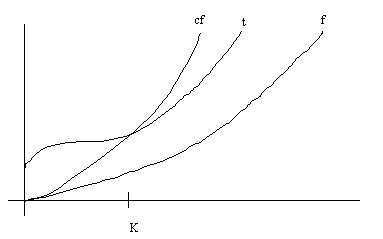Note that this only tells you what happens eventually; it says nothing about how `t` and `f` compare initially. The `O()` notation looks at behavior for large `n` (where running times are very important). The following definition says something even more exact about the growth of a running time function:

Definition: A running time function `t(n)` is `Theta(f(n))` provided that there are constants `c` and `d` such that `c * f(n) <= t(n) <= d * f(n)` for all `n` beyond some number `K`. (In other words, `t` is eventually bounded above and below by multiples of `f`.)

Of course, this is usually written with a Greek letter capital Theta, but we will write it out here. We read the statement that "`t(n)` is `Theta(f(n))`" as "`t(n)` is big-Theta of `f(n)`". We also sometimes say that "t(n) has order f(n)" or that "t(n) has the same order of magnitude as f(n)". In a picture we have something like the following: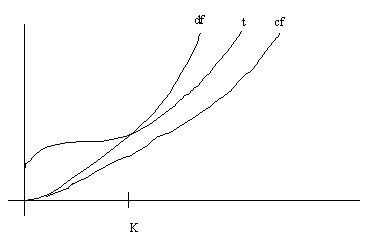Theorem: Any polynomial with leading term `c * n^k` is `O(n^k)`. In fact, it is `Theta(n^k)`.

We won't prove this, but will just look at examples of its use:

Function Growth
f(n) = 2*n^2 + n - 3 Theta(n^2)
g(n) = 5*n^3 + n^2 - 3*n + 14 Theta(n^3)

Roughly speaking, a `Theta(n^2)` function grows like the `n^2` function, at least for large values of n. For example, if you triple `n`, you would expect the function value to be about 3^2 = 9 times as much. A `Theta(n^3)` function grows roughly like the `n^3` function, at least for large `n`. Thus, if you triple `n`, you would expect the function value to be about 3^3 = 27 times as large. You might want to try graphing a `Theta(n^3)` function versus a `Theta(n^2)` one. Beyond a certain point the `n^3` one takes much more time. As `n` increases, the difference gets progressively worse.

Exponential functions (such as `t(n) = 2^n`) are incredibly bad for running time functions. They are known to have a worse order of magnitude than any polynomial function. An algorithm with exponential running time may take centuries to complete even for only moderately large values of `n`.

The following chart gives a comparison of some common orders of magnitude for running time functions. Note that lg(n) is used to mean log base 2 of n.

``````BEST                                            ||       WORST
-----------------------------------------------------------------
lg(n)   n   n*lg(n)   n^2   n^3   n^4  etc...   ||   2^n   n!
fairly good      ||   hopeless
||
``````

As a general rule, polynomial running times are fairly good, but exponential running times are totally impractical except for very small values on n.

### Searching an Array

#### Sequential Search

A very common operation is to search an array for a particular item. Check out the seqsrch.cpp example for one way to do this. The method used is commonly called a sequential search. This algorithm checks each item in the array, in sequential order, to see if it matches the one that we seek. The `SequentialSearch` function is short and easy to read. Note that it returns in the function name the array index of where it found the match or -1 if no match was found. The -1 can be used because it is not a valid array index.

What is the running time of this sequential search? Let's use `n` instead of `Count`, the number of data items in the array. Since the main task that gets repeated in the search is the `if` test that compares an item in the array with the `Target`, it makes sense to estimate the running time by counting the number of such data items tested. (This is sometimes called the number of probes of the array.)

In the best case, we find a match on the first probe. The first data item in the array is the one we wanted. In the worst case the number of probes is `n`; we have to check all of the data items in the array. In the average case the number of probes is approximately `n/2`. We can summarize the results as follows:

Case Running Time
Best Theta(1)
Average Theta(n)
Worst Theta(n)

The `LoadArray` function uses a random number generator to get data to place into the array. The first step is to "seed" the random number generator to a fairly random starting point by using the `srand` function. Here the starting point is based on the current system time as reported by the `time` function. Each time a new random integer is desired one just calls the `rand` function. The result is then scaled to be between 0 and 1000 in this program. Note that computer-based random number generators really generate pseudorandom numbers. The numbers appear to be random but in actuality are not quite random. In particular, the sequence of numbers generated form a long loop. Eventually the first number returns, with the second number coming after it, etc. Also, if the random number generator is called upon with the same seed it will produce exactly the same sequence of "random" numbers.

The `PrintArray` function in this example is interesting in that it shows one way to line up the output in columns. Here the `%` function (mod) is used to get the remainder after division by 15. When this remainder is 14 a newline is output. This has the effect of printing 15 items per line. The `setw` function is also used to get a field width of 5 for each integer printed. Read this carefully to see if you can follow it.

#### Binary Search

Another way to look for a target item in an array is by using a binary search. A binary search requires that all of the data be in order, typically in ascending order. (Ascending order means that as you follow the indices in order 0, 1, 2,... you get the data items in order such as 125, 200, 219,...). Look at the bsearch.cpp example.

This program fills `IntArray` with 100 numbers generated by `LoadArray` so as to be in increasing order. The first number is arbitrarily 1. Each succeeding number is generated by adding some random positive number to the previous number. This guarantees that the resulting data is in ascending order.

The `DoSearches` function allows the user to repeatedly look up an item in the array using binary search. Note how it uses the `tolower` function to make sure that the character in the `Reply` variable is in lower case. This makes testing for a yes answer easier because there is no need to test for both 'y' and 'Y'.

One way to visualize what functions call what other functions is to draw a structure chart. A structure chart for the bsearch.cpp program is shown below. It is like an organization chart for a company. It shows the `main` function at the top. Under `main` it shows the functions that are called from within `main`. Since `DoSearches` calls `BinarySearch`, that is shown with a line from `DoSearches` down to a box containing `BinarySearch`. This type of chart can be helpful in seeing the overall organization of "procedural programming". (Procedural programming consists in using functions to organize the program. Later you will study object-oriented programming, where objects are used to group data and the functions that operate on the data.)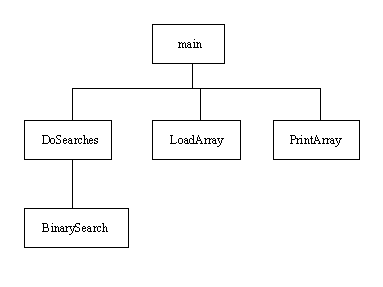The `BinarySearch` function has as parameters the array to be searched, the `Low` and `High` indices for the section of array to search (typically 0 to Count - 1), as well as the `Target` item for which to search. The function returns in the function name the index of where `Target` was found or -1 if it was not found.

Binary search uses a "divide and conquer" approach. You can see that it first finds a middle index, `Mid`, between `Low` and `High`. It then checks to see if the data item in the array at index `Mid` matches `Target`. If so, we are done; just return this index `Mid`. Otherwise, check to see if the data item at index `Mid` is smaller than `Target`. If so, we only need to check the section of the array to the right of `Mid`; anything to the left of `Mid` is only smaller and cannot possibly match. We arrange to search the right "half" of the array by changing `Low` to be `Mid + 1` and loop around to get the midpoint of that section, etc.

Similarly, if the data item at index `Mid` is larger than `Target`, we just need to search the left half of the array. We set up to do so by changing `High` to be `Mid - 1`. You can see the divide and conquer nature of the algorithm in how each probe of the array at a midpoint narrows the search to either the section to the right or left of the midpoint (or else finds a match). At each such probe we approximately divide the search space in half. For long arrays this is much faster than using a sequential search to methodically check every data item in the array!

It can be shown that binary search has `Theta(lg(n))` worst case and average case running time, where `n` is the number of data items in the array. Of course, the best case running time is `Theta(1)`, since we might find the desired match on the first probe of the array. In general, based on their average case behaviors, binary search is known as a `Theta(lg(n))` search, whereas sequential search is known as a `Theta(n)` search. As you can tell by graphing the functions `lg(n)` and `n`, the binary search will have a lot smaller running time for large values of `n`, and so is preferred when it is applicable. (Remember that the data in the array must be in order for binary search to be applicable. If it is not already in order, it could be sorted, but sorting is time-consuming. Sorting makes sense if we can sort the data once and then do a lot of searches through it, but if we keep adding new data items, for example, so that the array has to be sorted over and over, it is probably faster to skip the sorting and just use sequential search.)

The following drawings attempt to trace a binary search for a target of 23 in an array of 8 integers. The first probe of the array checks location 3 but does not find a match. Thus we narrow the search to the items to the left of index 3. The second probe checks location 1 but does not find a match. Thus we search the section to the right of index 1, a section with only 1 number in it. At this point, `Low`, `High`, and `Mid` all become 2. The third probe finds the 23. (Note that the item checked in each probe is underlined.)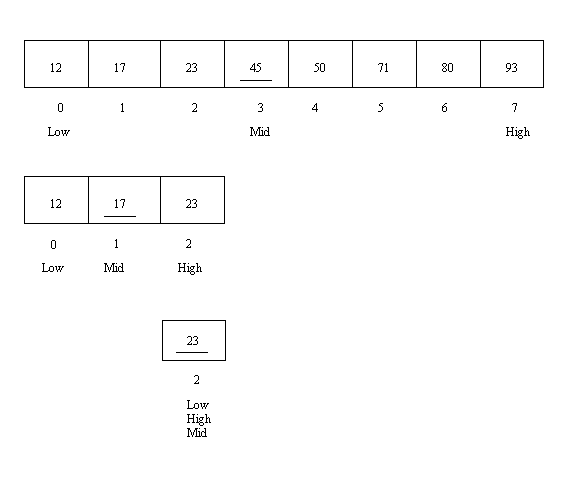What happens if we search for an item that is not in the array? This is called an unsuccessful search. For example, let's search the following array for 88.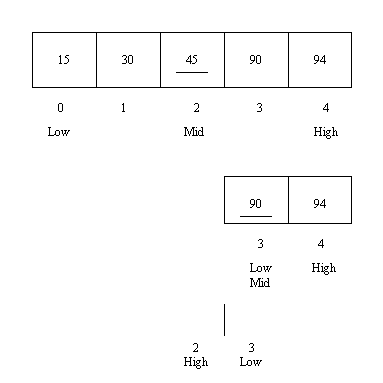Note that things proceed much like before. The first probe checks the 45, and the second probe checks the 90. Since the target 88 is smaller than the 90, we make `High` to be `Mid - 1`, which is 2. This is so that we can search the items to the left of index 3. However, there are no items to the left of index 3. The empty array section to be searched is drawn simply as a vertical line. The code detects this condition in the `while` loop test. Since `Low > High` the `while` loop ends, and the function returns a -1 to indicate that the 88 could not be found.

### Sorting an Array

#### Bubblesort

Another common operation is to sort the data in an array. We usually want to put the data into ascending order, but sometimes descending (backwards) order is used. Look at bubble.cpp for an example program using the well-known bubblesort algorithm.

Bubblesort is based on swapping out-of-order pairs of data items, in sequence, so as to bubble to the top of the array (if pictured vertically) the largest data item. That largest item is somehow marked as done and the same game of bubbling the largest (of the rest of the data items) to the top is played all over again, etc. Thus we get the largest at the top, then the second largest, then the third largest, etc.

Although our program sorts an array of integers, for the purpose of tracing the workings of bubblesort let's pretend that we have modified it to sort an array of characters. This leads to the following drawings, where each row (or "pass") is handled by the for loop and the succession of passes is handled by the while loop. The bracket in each drawing of the array shows the two data items that are being compared to see if they are out of order.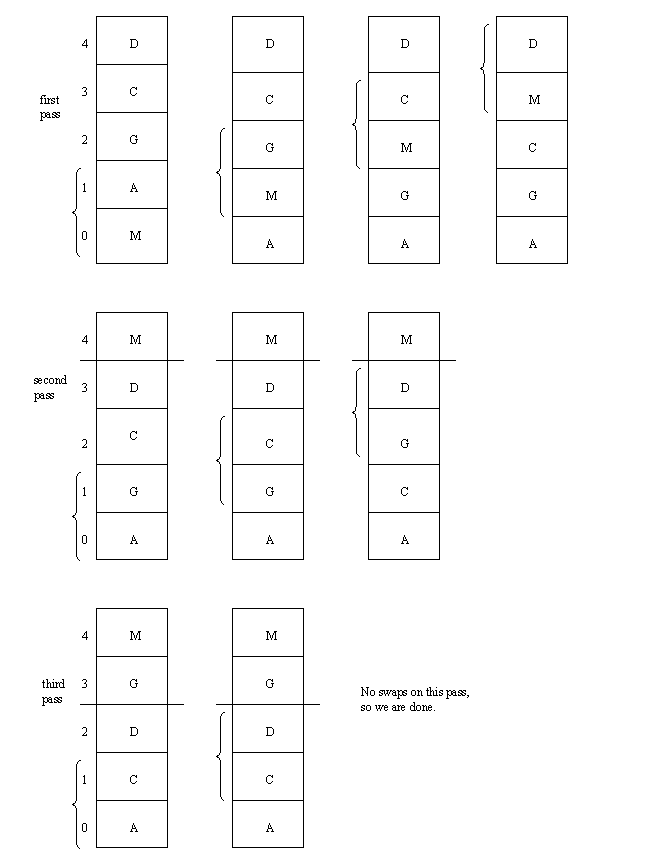This is a "smart" version of bubblesort in that it uses a flag called `Done` to keep track of whether or not any swaps were made on a pass. If no swaps are made on a pass that means that all of the items must have been in order, so that the sort can quit without doing any additional passes.

##### Inline Function

Note, too, the use of an inline `Swap` function. That means that the normal function call and return mechanism is not used. Instead a copy of the function code replaces the function call. This is faster and is appropriate for very short functions (so as to not add greatly to the program's length by inserting the same code everywhere where the function is called). An inline function can only be used after this section where it is defined; function prototypes are not used.

Let's use this program as another opportunity to draw a structure chart. Note that all of the functions other than `Swap` get called from the `main` function.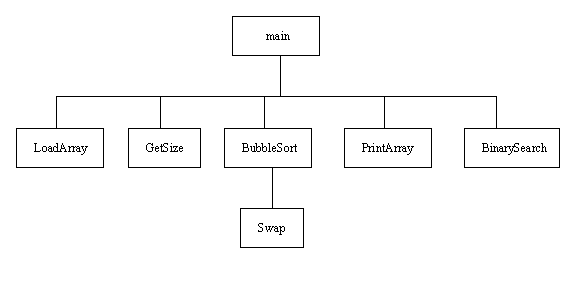Let's analyze the efficiency of bubblesort, especially in regard to its running time. This is usually estimated by counting the number of swaps of array items or by counting the number of key comparisons. In our simple example, the keys are the data items themselves, so that the key comparisons referred to are the greater than tests in the following `if`:

 `````` if (IntArray[k] > IntArray[k + 1]) { Done = false; // because we are making a swap Swap(IntArray[k], IntArray[k + 1]); } ``````

In the worst case (when the data is in descending order), every comparison will show that the data is out of order and thus result in a swap. In the worst case, then, the number of swaps equals the number of key comparisons. If the number of data items in the array is `n`, the number of swaps in the worst case is clearly `(n-1) + (n-2) + ... + 3 + 2 + 1 = n*(n-1)/2 = n^2/2 - n/2`, which is a `Theta(n^2)` function. Note that the running time for this sort grows much faster than the running time for either of our searches. Overall, bubblesort is known as a `Theta(n^2)` sort. Here is a summary:

Case # Swaps # Comparisons
Best None Theta(n)
Average Theta(n^2) Theta(n^2)
Worst Theta(n^2) Theta(n^2)

By the way, what is the best case? (It's when the data is already in order.) In such a case, bubblesort just does one pass with no swaps, so it is done with only `n-1` comparisons. Bubblesort, then, is very fast when the data is already in order (or probably when it is close to being in order).

As for memory space efficiency, bubblesort uses no appreciable extra memory space. There are no temporary arrays that consume a lot of space, etc.

#### Selection Sort

Another common sort routine is selection sort. Look at the select.cpp example program. It is essentially identical to the bubble.cpp program that we just looked at above, except that it sorts the array by using a selection sort, not a bubblesort.

The basic idea of our `SelectionSort` function is that it makes a pass through the array to find the index of the minimum item and then swaps that minimum item with the item at the bottom of the array. That bottom item is then marked as done. (This is shown in the drawing below by putting a solid line across.) Then the same game is played with the remaining items above that solid line: find the index of the minimum and swap it with the item at the bottom. By the time you reach the last picture in the drawing below, the smallest item is at index 0, the second smallest at index 1, etc. The only seemingly unchecked item is the one at the top, but it must be the largest by default. Thus the array has been sorted.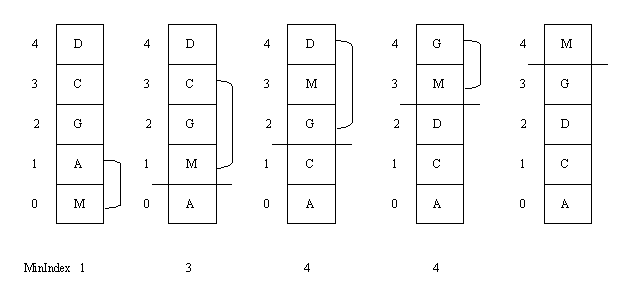In the drawing above, the index of the minimum is shown below each picture of the array. A selection sort can also be based on finding the index of the maximum on each pass. Note that our `SelectionSort` function may on occasion end up swapping an item with itself. If you want, you can add an extra condition to check that the indices are different before attempting a swap.

Let's estimate the running time of our selection sort as a function of the number of data items n in the array. As usual we can count the number of swaps or the number of key comparisons. The following chart shows the results:

Pass # Swaps # Comparisons
1 1 n-1
2 1 n-2
3 1 n-3
etc.
n-1 1 1
Totals n-1 (n^2)/2 - n/2

There is no best case or worst case, it always follows the same pattern. Overall, then, selection sort is obviously a Theta(n^2) sort just like bubblesort. Still, because the number of swaps is less than that of bubblesort, selection sort is probably a little bit faster than bubblesort. The following summarizes our results:

 # Comparisons Theta(n^2) Not good for large n # Swaps Theta(n) Very good, especially when swapping records

As with bubblesort, selection sort requires no appreciable amount of extra memory space.

### Character Arrays

An array of characters is a simple way to implement a string. The usual method is to end the string with a `NULL` character (ASCII 0) to mark the end. The NULL character can be written as the literal '\0', should you ever need it. A number of functions are available for working with `NULL`-terminated strings.

However, character array strings are not the safest. In particular, they make it easier to introduce buffer overflow flaws when copying strings. For this reason, the STL string class is usually the preferred way to handle strings.

Look at the program strings.cpp as a simple example of how to use the character array type of string. This program sets up `MaxString` as the maximum number of characters one of our strings can hold. Note that this includes the `NULL` character, so most people would say that the length of the string can be at most 11. Also, `StringType` is set up as a type name for any array of `MaxString` characters.

 `````` const int MaxString = 12; typedef char StringType[MaxString]; ``````

The most obvious method to try in reading a string from the keyboard is probably the following:

 `````` cin >> Message; ``````

There are several problems with this approach. First, the loading of characters into `Message` stops when a whitespace character such as a space is reached. That is a considerable problem if you are trying to read in a name such as "Jane Smith". Second, the newline that results from the user pressing the ENTER key at the end of whatever the person typed would still be sitting there in the input stream. If a second input statement is used, that input statement would see the input stream as having an empty string followed by a newline. To get around this second problem our program explicitly reads the newline (and does not store it anywhere) by using the following:

 `````` cin.get(); ``````

Although this fixes the second problem, the first problem remains. In addition, there is a third problem in that data entry of 12 or more characters results in the array being filled with characters without a `NULL` marking the end of the string. If more than 12 characters are entered, the program will try to write characters beyond the end of the array, possibly overwriting other data. This can lead to a run-time crash of the program.

Another way to read strings is to use the `getline` function as shown below.

 `````` cin.getline(Message, MaxString); ``````

The `getline` function by default reads until a newline is reached or until it has read `MaxString - 1` characters. Note that it is smart enough to leave room for the `NULL` character marking the end of the string and of course stores the `NULL` in the array. Thus `getline` reads in spaces without a problem. However, you do need to specify in the second parameter the maximum number of characters your string can hold (including the NULL). The first parameter is the string itself. Another advantage of `getline` is that it reads in and disposes of the newline character for you; no longer do we need to use a `get` to read the newline directly.

Note that even the `getline` method has some limitations. It cannot handle any string longer than 11 characters. The extra characters may end up being used for the next item of input or may be thrown away. The program may breeze right past the next input statement due to the extra characters. In any case, the results are not dependable if too many characters are entered.

The program ends by showing how to use the library functions `strcpy` and `strcat`. The `strcpy` function copies the string given by the second parameter into the first. The `strcat` function appends the string given by the second parameter to the string given by the first parameter. Note that `strcat` does not check if it runs off the end of the array. It is up to the programmer to verify that sufficient space is available in the string given as the first parameter. Our example program makes no such check. The `strcpy` function also does not check if it overflows the array that it is copying to. This can open up one's software to a buffer overflow attack, a well-known type of dangerous security flaw. A safer way to copy (and append) strings can be found in the STL string class. This class also provides the `at()` method for accessing individual characters within a string object with proper bounds checking instead. (The [] array subscript notation can be used to access individual characters of a string object but does not provide bounds checking.)

### Two-Dimensional Arrays

Arrays with dimension higher than two are possible, but will not be studied here. A two-dimensional array can be thought of as a table of data. The rows and columns are numbers (indexed) starting at 0 in both cases. A simple array of 3 rows and 4 columns that holds characters could be declared as follows:

 `````` char CharArray; ``````

One of the most important things to remember about two-dimensional arrays is that the row number always comes before the column number. This is true above where the array is sized, and it is true below where we arbitrarily place the letter 'G' into the location given by row 1, column 2.

 `````` CharArray = 'G'; ``````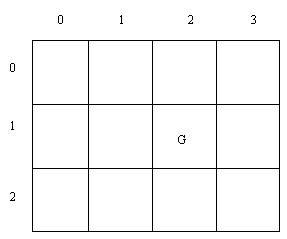#### A Two-Dimensional Array Example

Look at square.cpp as an example program containing a two-dimensional array. Note that a typedef is used to set up `ArrayType` as a type name for any 11 by 11 array of integers.

 `````` const int MaxSize = 11; typedef int ArrayType[MaxSize][MaxSize]; ``````

The `FillSquare` function contains the code to place values into the array. Note that it essentially amounts to the following two nested `for` loops:

 `````` for (Row = 0; Row < Size; Row++) for (Col = 0; Col < Size; Col++) { cout << "Enter a number: "; cin >> Value; Square[Row][Col] = Value; } ``````

Nested `for` loops are commonly used with two-dimensional arrays. The code in the `PrintSquare` function that prints out the array looks very similar to the above:

 `````` for (Row = 0; Row < Size; Row++) for (Col = 0; Col < Size; Col++) cout << Square[Row][Col]; ``````

Read through the rest of the program to see if you can understand both what it does and how it does it. Although this program appears not to do anything very useful, it turns out that subtracting from each item in a row the minimum for the row is used as part of the algorithm to solve certain problems in the field of operations research.

### Using An Index Array

An index array is an array of integers that is parallel to your array of data. It is sometimes used to speed up the sorting of the data, especially when the individual data items are large, such as long strings or large records. This is because it is somewhat time-consuming to swap large data items (say in our bubblesort studied above). With an index array, the numbers in the index array are swapped instead of the items in the data array. Since integers are rather small, it is fast to swap them. The only complication is that all accesses to items in the data array must be done via the index array. For example, to print out the data after the sort is finished, one would use something like the following:

 `````` for (k = 0; k < 5; k++) cout << A[Index[k]]; ``````

The following drawing attempts to show the index array and data array `A` before the bubblesort is done, after one swap was done, and after the entire sort has completed. Note that the data in `A` is never changed; all changes take place in the index array.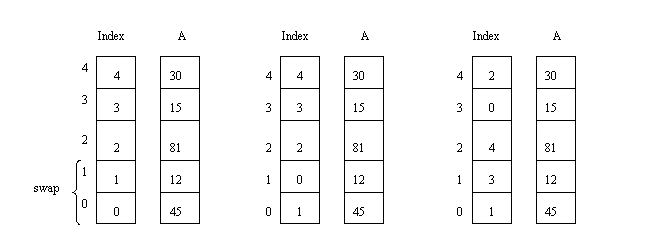Of course, the code for our bubblesort also has to be modified. The essential change is in the `if` test that decides whether or not to swap. As shown below, it must now access the data array `A` via the index array and must also swap indices in the index array. A complete program is left as one of those proverbial exercises for the reader.

 `````` if (A[Index[k]] > A[Index[k + 1]]) { Done = false; Swap(Index[k], Index[k + 1]); } ``````

### Related Items

 Basics of Arrays Introductory Topic. Vectors (in STL)Advanced Topic. Strings (in STL)Advanced Topic. Professional Programming: Issues and ToolsAdvanced Topic.
Back to the main page for Software Design Using C++

Author: Br. David Carlson with contributions by Br. Isidore Minerd
Last updated: February 18, 2022
Disclaimer